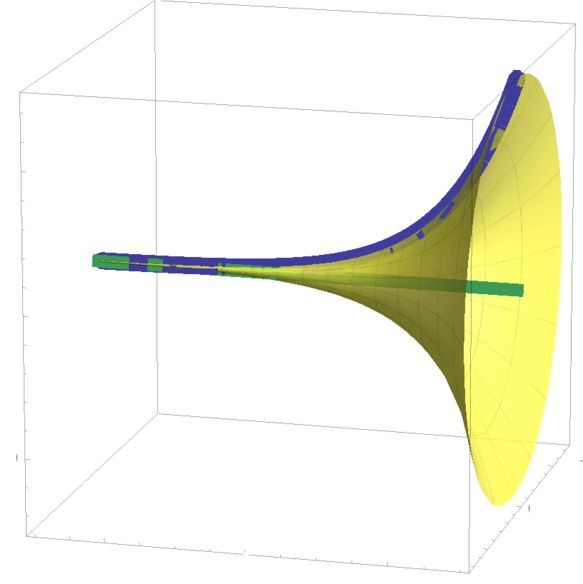# Looks Like Gabriel's Horn To Me

Calculus Level 5An infinitely long cone can be generated by revolving the following curve about the $x$-axis.

$y = e^{x}, \quad x < 0$

If the ratio of volume to lateral surface area of the cone is $\beta$, then what is $\lfloor 10^4 \times \beta \rfloor$?

Notation: $\lfloor \cdot \rfloor$ denotes the floor function.

×